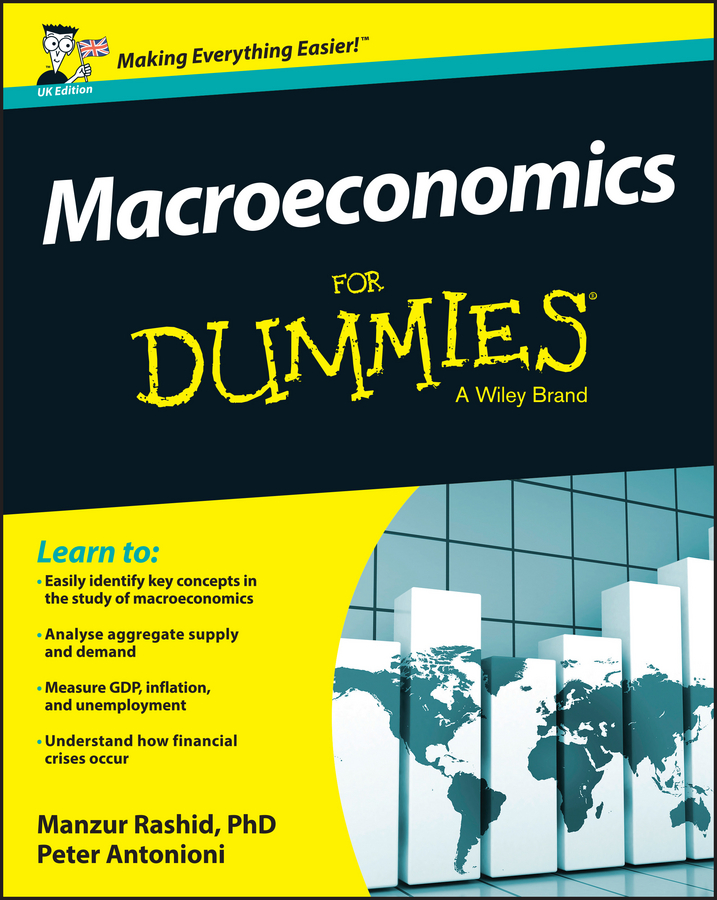##### Macroeconomics For Dummies - UKCurious what a modern dollar was worth 30 years ago? If so, then you are interested in calculating inflation. Inflation is the consistent increase in the prices of goods and services in an economy over time. Why does a brand new car cost so much more today than it did 20 years ago? Again, the answer can be found with inflation.

The process to calculate inflation using the Consumer Price Index is not a complicated task. You will find out how to make these calculations manually below. However, the easiest method is to simply use an inflation calculator. Free versions are easily found on the internet. Follow these steps to find an inflation calculator:

1. Open your favorite web browser.
2. Search for inflation calculator.
3. Enter your dates and dollar amount into the inflation calculator of your choice.
4. Hit Calculate.
The inflation calculator will do the math for you.

If you want to calculate the inflation manually, you will first need to visit the Consumer Price Index (CPI) site.

Make note of the number listed on both dates that you are interested in. For example, if you’d like to know how much a dollar from March of 2015 was worth in 1980, you would make note of the CPI number listed in March of 1980 and March of 2015. Let’s use those numbers to make the calculations. For this example, those numbers are 80 and 236, respectively.

If you inspect the CPI index, you will notice that the numbers actually appear as 80.100 for March of 1980 and 236.119 for March of 2015. For most examples, it is acceptable to round to the nearest whole number. For the purposes below, you can use 80 for March of 1980 and 236 for March of 2015.

1. Calculate the increase. To do this, subtract the original CPI from the final CPI.

In this example, you would subtract 80 from 236 (236–80=156) for an increase of 156.

2. Divide by the original. This means that you should divide your increase in CPI by the original CPI.

In this example, you would calculate 156÷80. After you perform the calculation, your answer is 1.95.

3. Convert your answer to a percent.

To do this, simply multiply 1.95 by 100 (1.95×100=195). This results in 195%.

What does this mean? It means that between 1980 and 2015, the price of items increased by 195%.

4. Multiply by the dollar amount.

In this example, you are just curious about how much a dollar in 1980 is worth in 2015, so you would use the number 1 as the dollar amount.

5. Multiply 1 by 1.95 (1×1.95) to get the amount you need to add to your original dollar amount of \$1.

1×1.95=1.95 or \$1.95.

Add that to your original \$1 (1+1.95) and you get a total of \$2.95.

Your 1980 dollar is worth \$2.95 in 2015.

Now, let’s try another example. Using the concept of a new car, let’s calculate how much a \$5,000 car bought in 1970 would cost you in 2010. For this example, let’s use July of 1970 and July of 2010. Follow the steps below.

1. First, check the Consumer Price Index (CPI) site for the respective numbers in July of 1970 and July of 2010.

These numbers are 39 and 218, respectively.

2. Calculate the increase by subtracting 39 from 218 (218–39). These have been rounded to the nearest whole number.

This results in 179.

3. Divide the increase by your original CPI.

So, divide 179÷39. This results in 4.59.

4. Convert your answer to a percent.

To do this, multiply 4.59×100. This results in 459 or 459%. Now, you know inflation has risen by 459%.

5. Next, multiply by the dollar amount by the increase of 4.59.

Multiply 5,000×4.59. This gives you 22,950.

6. Add the amount to your original number.

This would be 5,000+22,950. Performing this calculation gives you 27,950.

A car costing \$5,000 brand new in 1970 would cost you \$27,950 in 2010.